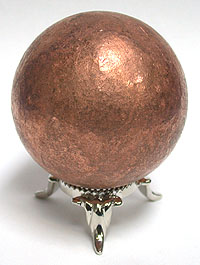# Professor $\mathfrak{X}$ and his experiments! -1Professor $\mathfrak{X}$ is a very ingenious scientist and researcher.

Once Professor $\mathfrak{X}$ was doing some experiments in his laboratory related to heat and thermal expansion. He took a metal ball and heated it until its temperature increased by $30\ ^{\circ}\text{C}.$ Then he found that the volume of the metal ball increased by $0.18\%$.

Assuming isotropic behavior of the metal ball, calculate the coefficient of linear expansion of the metal and help Professor $\mathfrak{X}$ in his research.

×